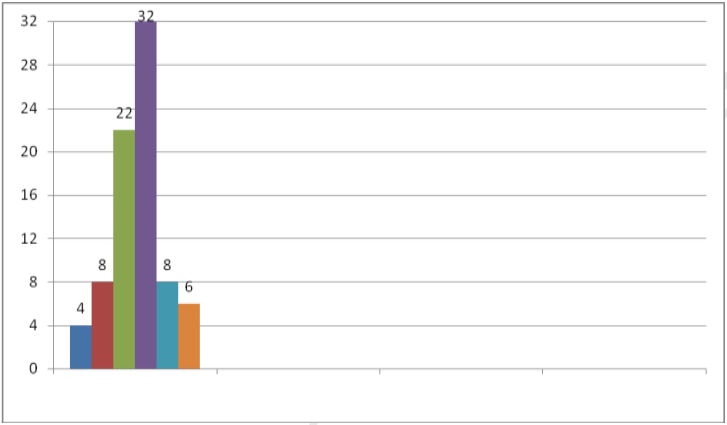# MCQ Questions for Class 8 Maths Chapter 5 Data Handling with Answers

Free PDF Download of CBSE Maths Multiple Choice Questions for Class8 with Answers Chapter 5 Data Handling. Maths MCQs for Class 8 Chapter Wise with Answers PDF Download was Prepared Based on Latest Exam Pattern. Students can solve NCERT Class 8 Maths Data Handling MCQs Pdf with Answers to know their preparation level.

## Data Handling Class 8 Maths MCQs Pdf

1. The width or size of the class interval 30-40 is:
a) 10
b) 30
c) 40
d) 70

Explanation: Width of class interval = upper class limit-lower class limit

= 40-30

= 10

2. If a coin is flipped in the air, what is the probability of getting a tail?
a) 0
b) ½
c) 1
d) 2

Explanation: A coin has two sides, Head and tails.

Hence, probability = Number of possible outcomes/Total number of outcomes

We know, Tail is present on one side of a coin and in total there could be two outcomes H&T

Hence, Probability = ½

3. A bag has 4 red balls and 4 green balls, what is the probability of getting a red ball randomly?
a) 1/4
b) 1/8
c) 1/2
d) 0

Explanation: Total number of balls in bag = 4+4 = 8

Total number of red balls = 4

Probability of getting a red ball = 4/8 = ½

4. If a die is thrown in air, the probability of getting even numbers is:
a) ½
b) ⅙
c) ⅓
d) ⅔

Explanation: A die has six sides each consisting numbers from 1 to 6.

Number of even numbers in die = 3 (2,4,6)

Total possible outcomes = 6

Probability = 3/6 = 1/2

5. Pictorial representation of data using symbols is known as:
a) Bargraph
b) Pictograph
c) Piechart
d) None of these

6. Double bar graphs display ______ sets of data simultaneously.
a) Four
b) Three
c) Two
d) No

7. _________ gives the number of times a particular entry occurs.
a) Organisation of data
b) Collection of Data
c) Representation of Data
d) Frequency distribution table

8. In grouped data, each of the group is called:
a) Class interval
b) Collection of data
c) Frequency
d) Grouped frequency distribution

9. If 20-30 is the class interval of grouped data, then lower class limit is:
a) 50
b) 30
c) 20
d) 10

10. The difference between the upper class limit and lower class limit of a class interval is called:
a) Frequency
b) Width
c) Grouped data
d) Ungrouped data

Frequency distribution of daily income of 550 workers of a factory is given bellow
Read the table given above and answer the following questions (Q1 – Q5)
11. What is the size of class intervals?
(a) 24
(b) 25
(c) 26
(d) 15

12. Which class has the highest frequency?
(a) 200-225
(b) 300-325
(c) 175-200
(d) 150-175

13. Which class has the lowest frequency?
(a) 100-125
(b) 300-325
(c) 175-200
(d) 150-175

14. What is the upper limit of the class interval 250-275?
(a) 250
(b) 275
(c) 25
(d) 525

15. Which two classes have the same frequency?
(a) III & IV
(b) I & II
(c) II & V
(d) V & VI

The number of hours for which students of particular class watched television during holidays is shown through the graph given below:16. For how many hours did the maximum number of students watch TV?
(a) 4-5 hrs
(b) 6-7 hrs
(c) 3-4 hrs
(d) 2-3hrs

17. How many students watched TV for less than 4 hrs?
(a) 12
(b) 34
(c) 4
(d) 8

18. How many students spent more than 5 hrs in TV watching?
(a) 14
(b) 0
(c) 6
(d) 8

19. For how many hours did the minimum number of students watch TV?
(a) 2-3 hrs
(b) 6-7 hrs
(c) 1-2 hrs
(d) 3-4hrs

20. How many students spent less than 5 hrs in TV watching?
(a) 34
(b) 32
(c) 8
(d) 66

Adjoining pie-chart gives the expenditure (in %age) on various items and savings of a family during a month .
Study the given pie chart and answer the following questions (Q11-Q15)21. On which item the expenditure was maximum?
(a) food
(b) education
(c) others
(d) transport

22. On which item the expenditure was minimum?
(a) food
(b) education
(c) others
(d) transport

23. Expenditure on which item is equal to total savings of the family?
(a) food
(b) education
(c) others
(d) transport

24. If the monthly savings of the family is Rs 3000, What is the monthly expenditure on cloths?
(a) 3000
(b) 2000
(c) 2500
(d) 1000

25. What is the monthly expenditure on education for children?
(a) 3000
(b) 2000
(c) 2500
(d) 1000

Numbers 1 to 10 are written on ten separates slips (one number on one slip ), kept in a
box and mixed well. One slip is chosen from the box without looking in to it .Answer the
following questions (Q16-Q20)

26. What is the probability of getting a number 6?
(a) 1
(b) 0
(c) $$\frac{1}{10}$$
(d) $$\frac{1}{2}$$

27. What is the probability of getting a number less than 6?
(a) 1
(b) 0
(c) $$\frac{1}{10}$$
(d) $$\frac{1}{2}$$

28. What is the probability of getting a number greater than 6?
(a) 1
(b) 0
(c) $$\frac{1}{10}$$
(d) $$\frac{2}{5}$$

29. What is the probability of getting a 1-digit number?
(a) 1 (b) 0
(c) $$\frac{1}{10}$$
(d) $$\frac{9}{10$$

30. What is the probability of getting an even number?
(a) 1
(b) 0
(c) $$\frac{1}{10}$$
(d) $$\frac{1}{2}$$